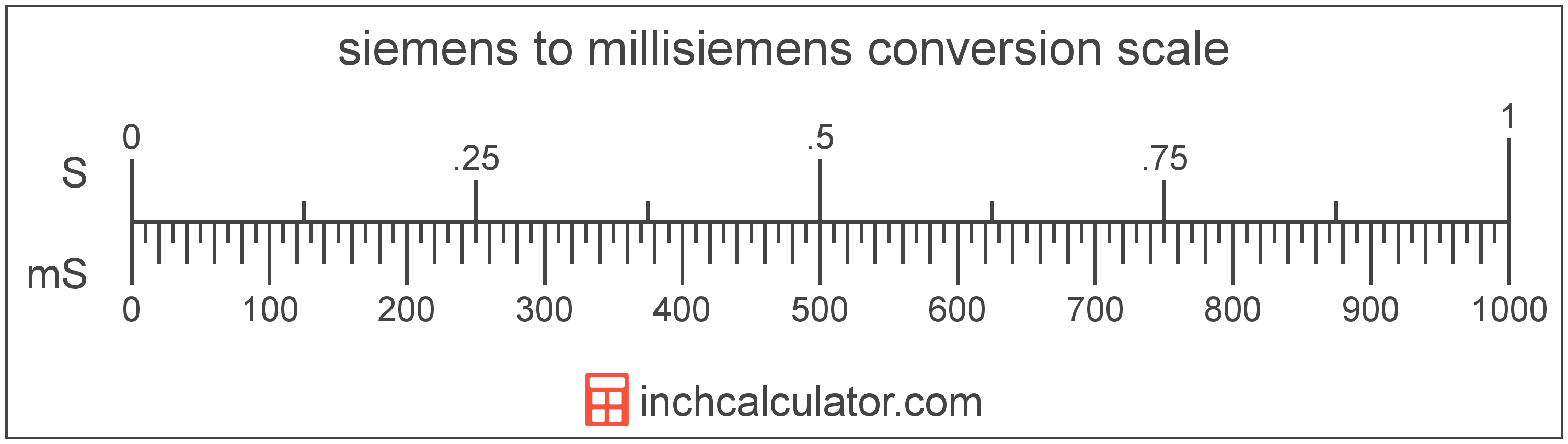# Siemens to Millisiemens Conversion

Enter the electrical conductance in siemens below to get the value converted to millisiemens.

Results in Millisiemens:1 S = 1,000 mS

## How to Convert Siemens to Millisiemens

To convert a siemens measurement to a millisiemens measurement, multiply the electrical conductance by the conversion ratio.

Since one siemens is equal to 1,000 millisiemens, you can use this simple formula to convert:

millisiemens = siemens × 1,000

The electrical conductance in millisiemens is equal to the siemens multiplied by 1,000.

For example, here's how to convert 5 siemens to millisiemens using the formula above.
5 S = (5 × 1,000) = 5,000 mS### How Many Millisiemens are in a Siemens?

There are 1,000 millisiemens in a siemens, which is why we use this value in the formula above.

1 S = 1,000 mS

## Siemens

One siemens is the electrical conductance equal to an increase in current of one ampere for every increase in electrical potential difference of one volt. The siemens is the SI defined unit equal to the mho, and is the reciprocal of the resistance in ohms.

The siemens is the SI derived unit for electrical conductance in the metric system. Siemens can be abbreviated as S; for example, 1 siemens can be written as 1 S.

## Millisiemens

The millisiemens is the SI defined unit equal to the millimho. One millisiemens is the electrical conductance equal to 1/1,000 of a siemens, which is equal to one ampere per volt.

The millisiemens is a multiple of the siemens, which is the SI derived unit for electrical conductance. In the metric system, "milli" is the prefix for 10-3. Millisiemens can be abbreviated as mS; for example, 1 millisiemens can be written as 1 mS.

## Siemens to Millisiemens Conversion Table

Siemens measurements converted to millisiemens
Siemens Millisiemens
0.001 S 1 mS
0.002 S 2 mS
0.003 S 3 mS
0.004 S 4 mS
0.005 S 5 mS
0.006 S 6 mS
0.007 S 7 mS
0.008 S 8 mS
0.009 S 9 mS
0.01 S 10 mS
0.02 S 20 mS
0.03 S 30 mS
0.04 S 40 mS
0.05 S 50 mS
0.06 S 60 mS
0.07 S 70 mS
0.08 S 80 mS
0.09 S 90 mS
0.1 S 100 mS
0.2 S 200 mS
0.3 S 300 mS
0.4 S 400 mS
0.5 S 500 mS
0.6 S 600 mS
0.7 S 700 mS
0.8 S 800 mS
0.9 S 900 mS
1 S 1,000 mS